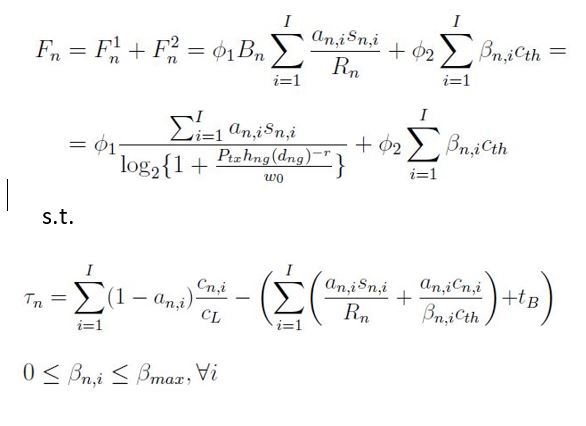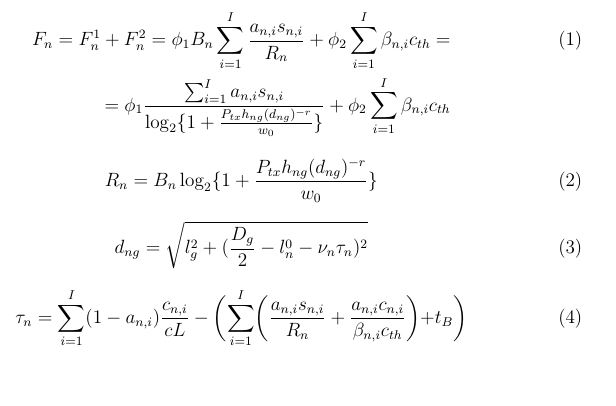# How to write this formula into CVX?

Hey guys,

I have to solve an optimization convex problem but I have problems to replicate the formula in cvx.
The objective function to be minimized is:I represents the number of tasks, a_n, s_n, c_n are all inputs of the problem. I have to find a set of beta_n_i that minimizes the objective function but I’m unable to write the expression, my problem is with tau_n, because it is a constraint of the problem but it depends itself on beta_n,i.

The tau_n constraint is a nonlinear equality constraint in terms of beta_n_i, which would be non-convex.

However, if you make 1/beta_n_i (call it, inv_beta_n_i, or whatever you want) the optimization (CVX) variables instead of beta_n_i, then presuming the relevant parameters have the needed sign, that constraint looks like it would be an affine equality constraint, and the objective looks like it could be written using `inv_pos`, that is, unless there is hidden stuff lurking there (why even bother with the first term of the objective function if it is constant?).

Thank you for you speedness in providing assistance.
First of all, everything is granted to be convex due to dedicated math proofs.
I’m trying to express better my issue.The point is I have something sort of “recursive” in terms of definitions. The objective function to be minimized is espressed in eq. 1, but the problem is about the definition of parameter d_ng reported in eq. 3 function of tau_n.
tau_n is defined according eq. 4 which is function of beta_n and R_n. In particular this last one is defined in eq. 2 , function of d_ng (function of tau_n itself). So finally the problem is about having defined a function by itself and I’m not able to report everything on a single line, in order to express it in CVX. Is there any particular procedure?

I don’t know where you found this problem, but I put to you the responsibility of understanding it. I don’t know what all those symbols are, which of then are provided as inputs, etc. That is for you to understand.

Do equations (2) and (3) merely provide formulas for deriving some “input” parameters in terms of others? If so, they are not really part of the optimization problem, but just calculations which should be made prior to starting the optimization. However, equation (4) appears to be a constraint of the optimization problem. I am presuming that tau_n is input, which allows calculation of the Left Hand Side of (3). If that is not the case, you need to figure put what is the case, and clearly explain it to us.

Whether you realized it or not, if you had gotten past the concerns you stated, you would have been confronted with the matter which I pointed out, and provisionally solved, contingent on there not being “hidden” stuff.

In your CVX program, all input data (i.e., everything except for CVX variables and expressions involving them) must have numerical values prior to their first use in CVX objective function or constraints. That means the supplementary calculations should be done before parameters determined in terms of them are used in CVX.

It is your responsibility to tell us what the optimization variables are, and what the inputs are. it is your responsibility to figure out how to come up with the inputs you need for the problem.

I apologize for having omitted several assumptions. The optimization problem that I presented here concerns a model of a scientific article that I am trying to implement. More in detail:
phi1, phi2, a_n, s_n, c_th, w0, Ptx, hng, r, lg, Dg, ln0, v_n, c_L, t_B, c_n are all constants at the input of the problem.
The objective function to be minimized is F_n. In particular we want to find the set of beta_n that minimize F_n; the constraints are those declared in the first image of the topic.
My problem arises from the way tau_n is defined, which depends on beta_n and R_n which in turn depends on d_ng which depends on tau_n. There is this sort of recursion that I can’t implement.

I interpret that tau_n is an input. Equation (4) is a constraint on the optimization variables beta_n_i. That constraint requires the value of tau_n to be provided as an input to the optimization problem. So is tau_n an input or an optimization variable?

Everything in equations (1) and (4), other than beta_n_i, needs to be provided or determined as an inpot to the optimization problem.Presumably, you need input values of everything on the right-hand sides of (2) and (3) in order to calculate their left-hand sides. At that point, you better have numerical values for everything in (1), (4) ,and the bound constraint (5?), other than beta_n_i.

Perhaps you need to restudy the article with this in mind.

Dear Mark, thank you for your support. I tried to follow your advice, I considered tau_n as an input of the optimization problem. I realized the following script, with the section in CVX:

clear all
close all

Dg = 600;
lg = 20;
Bmax = 2010^6;
r = 2.5;
hng = 10^-3;
cL = 2
10^8;
cth = 810^8;
phi_1 = 1
10^(-6);
phi_2 = 310^-6;
tB = 0.1;
Ptx = 1;
Tmax = 6;
I = 20;
betamax = 0.8;
betamin = 0;
w0 = 10^-9;
toff = 0:0.2:3.8;
cn = 40
10^6 + 8010^6 . rand(1,I);
sn1 = 210^6 + 410^6 .*rand(1,I);
sn = sort(sn1,‘descend’);
epsn = 3;

an = zeros(1,I);
app = 0;
cn_ordinato = sort(cn, ‘descend’);

for i = 1:I
app = app + cn_ordinato(i)/cL;
controllo = Tmax - app;
if (controllo>=epsn)
an(i) = 0;
else
an(i) = 1;
app = app - cn_ordinato(i)/cL;
end
end

%%%%%%%%%%%%%%%%%%%%%%%%%%%%%%%%%

ln0 = 250;
vn = 22.2;

for i=1:length(toff)
dngin = sqrt(lg^2+(Dg/2 - ln0 - vn * toff(i)).^2);
Rn = Bmax * log2(1+(Ptx * hng * dngin.^(-r)/w0));

cvx_begin
variable x(I)
maximize( phi_1* Bmax * (sum(an.sn)./Rn) + phi_2 * sum(xcth) )
subject to
betamin <= x <= betamax;
toff(i) = sum((1-an).*(cn_ordinato./cL)) - (sum(((an.*sn)./Rn)+((an.cn_ordinato)./(xcth))+tB));
cvx_end
Fn(i)= cvx_optval;
end

But I have this error:
Error using .* (line 46)
Matrix dimensions must agree.

Error in ./ (line 19)
z = times( x, y, ‘./’ );

Error in provacvx (line 73)
toff(i) = sum((1-an).*(cn_ordinato./cL)) - (sum(((an.*sn)./Rn)+((an.cn_ordinato)./(xcth))+tB));

Can you help me to fix it?
I’ll let you figure out what the dimension error is. If instead of declaring `x` as a variable, you assign it a numerical value as an n by 1 vector, then the right hand side of that “constraint” needs to evaluate without error in MATLAB. I don’t know if it does. But in any event, you are making an assignment using `=`, when you need to make a constraint using `==`. And perhaps doing that as an illegal expression array (see http://cvxr.com/cvx/doc/basics.html#assignment-and-expression-holders ) is triggering an error.